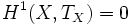# Matsushima-Shimura theorem

## Statement

Let$X$ be an irreducible compact quotient of a polydisc. Then:

•$H^1(X,T_X) = 0$
• As a corollary,$X$ is locally rigid as a complex manifold

## Relation with other results

### Calabi-Vesentini theorem

This draws the same conclusions from slightly more relaxed hypotheses.

Further information: Calabi-Vesentini theorem